# 【TS】快速上手（二）型別宣告

theme: fancy highlight: tomorrow-night-blue

「這是我參與11月更文挑戰的第25天，活動詳情檢視：2021最後一次更文挑戰

• 官網：https://www.typescriptlang.org/
• 中文網：https://www.tslang.cn/ （貌似很久沒維護了）
• 掘金小冊： https://juejin.cn/book/6844733813021491207
• 書籍：《深入理解TypeScript》 https://jkchao.github.io/typescript-book-chinese/
• B站尚矽谷影片：https://www.bilibili.com/video/BV1Xy4y1v7S2

# 1. 起步

• 型別宣告是TS非常重要的一個特點
• 通過型別宣告可以指定TS中變數（引數、形參）的型別
• 指定型別後，當為變數賦值時，TS編譯器會自動檢查值是否符合型別宣告，符合則賦值，否則報錯
• 簡而言之，型別宣告給變數設定了型別，使得變數只能儲存某種型別的值

```ts // 宣告一個變數 let 變數: 型別

// 宣告一個變數並給其賦值 let 變數: 型別 = 值

// 宣告一個函式 function yk(引數: 引數型別): 函式返回值型別{ //... } ``` 這裡我們可以看到，就是在變數後面就一個冒號，然後標識其型別就可以了

# 2. 型別

## 2.1 變數

```ts let n: number = 1 n = 3 n = '1' // 編輯器報錯，不能將字串賦值給number型別的變數```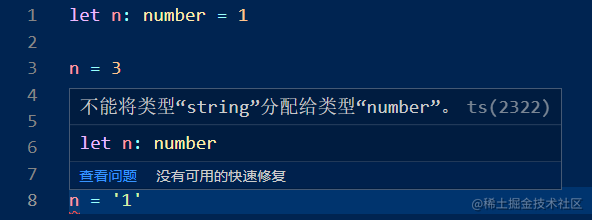## 2.2 自動型別判斷

• TS擁有自動的型別判斷機制
• 當對變數的宣告和賦值是同時進行的，TS編譯器會自動判斷變數的型別
• 所以如果你的變數的宣告和賦值時同時進行的，可以省略掉型別宣告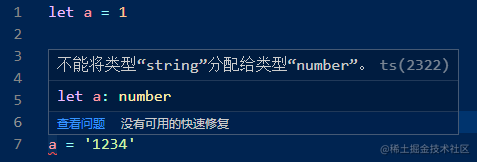## 2.3 函式

```js function sum(a, b){ return a + b }

console.log(sum(1, 2)) // 3

console.log(sum(1, '2')) // '12' ```

```ts function sum(a: number, b: number): number{ return a + b }

console.log(sum(1, 2)) // 3 ```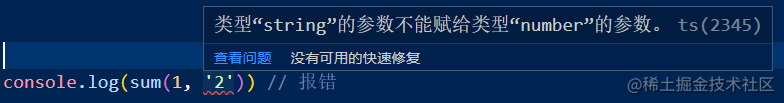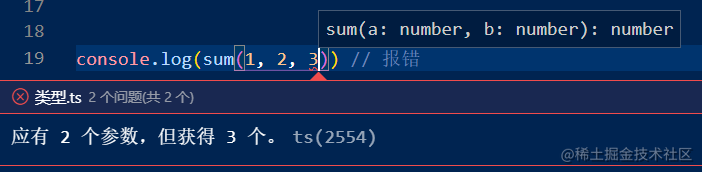## 2.4 型別詳解

### void

```js function fn(): void { return }``` 返回一個`undefined`

```js function fn(): void { return undefined }```

```js function fn(): void { return null }```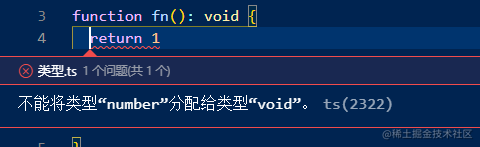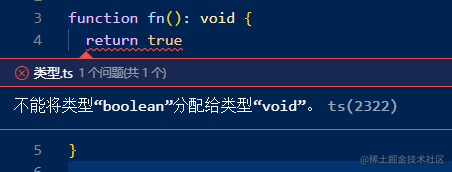### any

`any` 表示的是任意型別，一個變數設定型別為 `any` 後，相當於對該變數關閉了TS的型別檢測。

```ts let a let b: any a = 1 a = '1' b = '1' b = 1

let c:string = '123' c = b ```

`any`型別是多人協作專案的大忌，很可能把Typescript變成AnyScript，通常在不得已的情況下，不應該首先考慮使用此型別。

### unknown

`unknown``any`的區別就是，將`unknown`型別變數賦值給其他任意型別的變數時，會報錯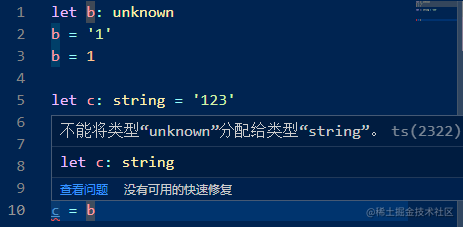```ts if (typeof b === 'string') { c = b }```

```ts c = b as string```

```ts c = <string>b```

### never

`never` 表示永遠不會返回結果

```js function fn(): never{ throw new Error('報錯了！') }```

### 物件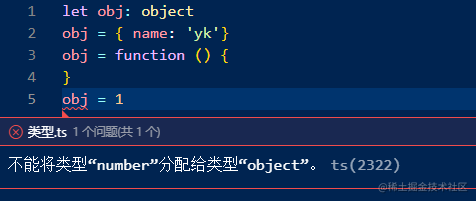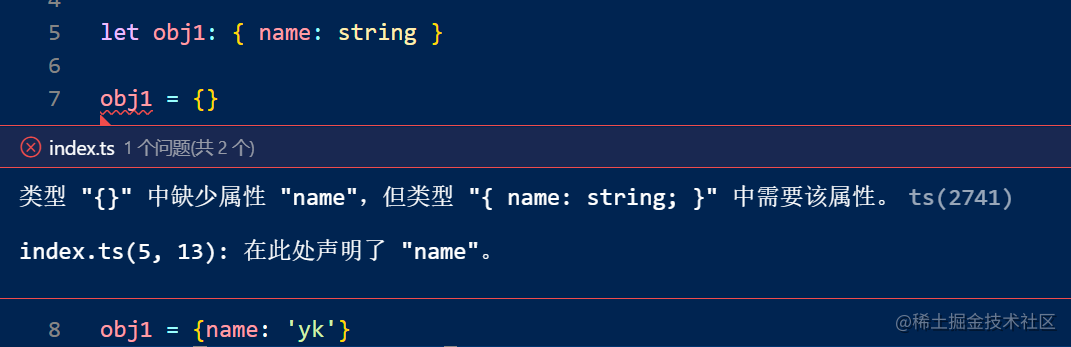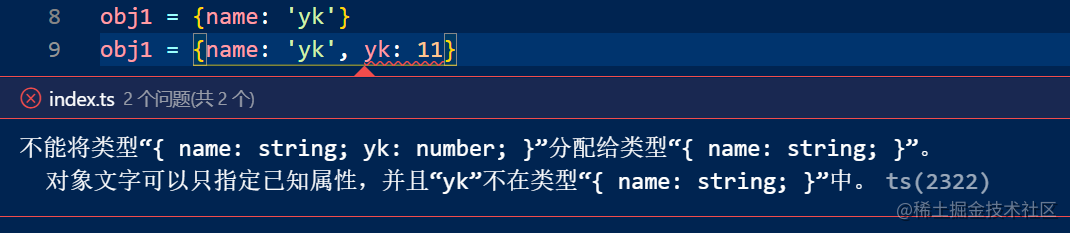```ts let obj: {name: string, age?: number} obj = {name: 'yk', age: 18} obj = {name: 'yk'}```

```ts // 定義物件結構 let obj: { name: string, [propName: string]: unknown }

obj = {name: 'yk', age: 18, gender: 'male'} ```

### 函式

```js let fn: Function```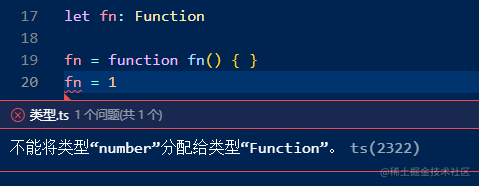```ts // 定義函式結構 let fn: (a: number, b: number) => number```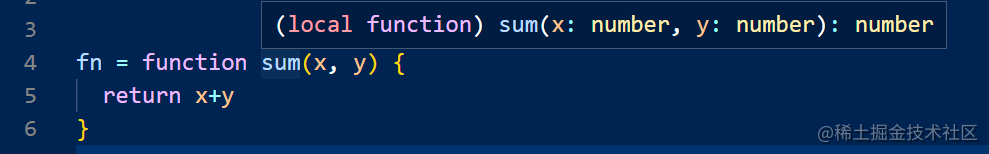### 陣列

```ts let list: Array<number> = [1, 2, 3] let list2: Array<string> = ['y', 'k']```

### 元組 Tuple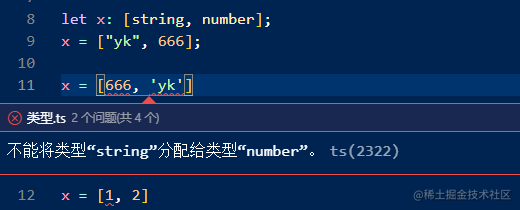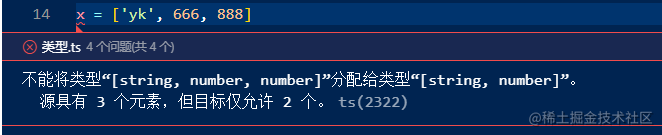### 字面量

```js let a: 10 a = 10```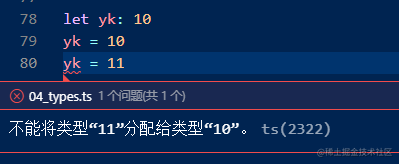## 2.6 類型別名

```ts type myType = 1 | 2 | 3 | 4 | 5 let i: myType let j: myType let k: myType

k = 2 ```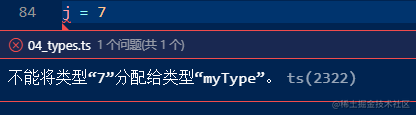## 2.7 總結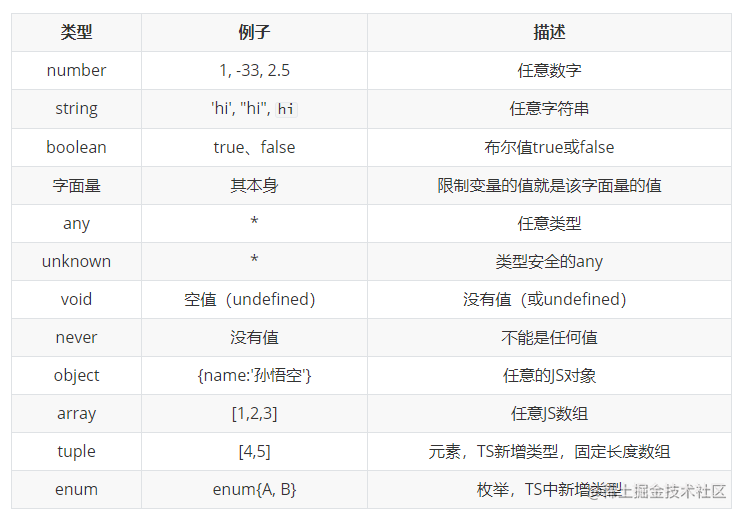# 3. 型別斷言

```ts let someValue: unknown = "this is a string"; let strLength: number = (someValue as string).length;```

```ts let someValue: unknown = "this is a string"; let strLength: number = (<string>someValue).length;```